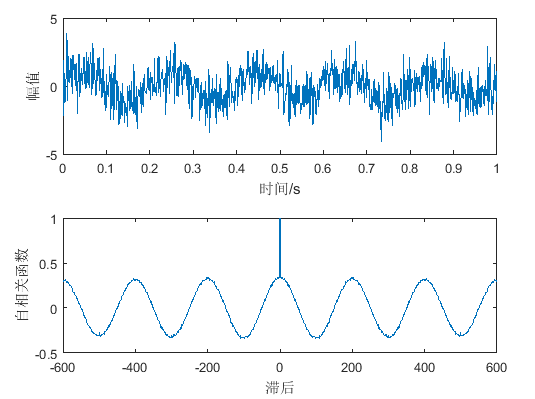# 相关函数的意义和工程应用

## 摘要

小虎在这里介绍了相关函数的意义和工程应用，工程应用以提取受噪声干扰的周期信号为例，并用MATLAB进行仿真。

## 什么是相关函数

相关函数(correlation function)是用来衡量两个信号的相关程度。相关函数又分为自相关函数、互相关函数和协方差函数。这里仅介绍在测试技术中较常见的两种（前两种）。

### 自相关函数

自相关函数(autocorrelation function)是用来衡量统一信号在不同时刻取值的相关程度。通过这种分析，可以分析出信号中的噪声并加以去除；或者从畸变的波形中分离出基波和谐波等。可以将时移前后的信号当成两个信号分析。这是仿真理解demo
$R_x(\tau)=\lim \limits_{T \to \infty } \frac{1}{T} \int_0^Tx(t)x(t+\tau)dt$

### 互相关函数

互相关函数(crosscorrelation function)用来衡量两个信号之间的相关程度和取值依赖程度。对于一个理想的测试系统的输入输出信号求相关函数，测试结果完美的情况下，互相关函数取最大值时的$\tau$时为等于系统的滞后时间，因为这说明信号并没有损失或收到噪声干扰，给输入信号加上的延时$tau$后与输出的信号一模一样，所以相关程度为完全相同。这是仿真理解demo
$R_{xy}(\tau)=\lim \limits_{T \to \infty } \frac{1}{T} \int_0^Tx(t)y(t+\tau)dt$
另有性质之一$R_{xy}(-\tau)=R_{yx}(\tau)$，所以当$y(t)$比较复杂时可以采用这种方法转换为对$x(t)$时延来计算。

## 相关函数提取周期信号原理

对机器进行噪声诊断时，噪声通常由随机、大量且大小近似相等影响因素叠加而成。随机噪声的自相关函数将出现规则的周期信号，幅值一般比正常噪声的幅值要大。当将变速箱各个机轴的转速化成频率与自相关函数波动频率比较，可以确定机械轴的好坏。或者利用自相关函数分析去除噪声得到周期函数的周期。

## 具体例子——MATLAB仿真示例

提取受到噪声干扰的周期函数$x=sin(w_0t)$的自相关函数,分析原周期函数的周期。

### 物理意义

结果如下图。为了便于计算，将$w_0=2\pi f_0$计算。• 可以看到，每过一个周期，原周期函数重合，相关程度最高；每过半个周期，原周期函数互为相反数，相关程度为负的最大，这里我设的函数周期正好是0.2s（即200ms），可以很好的从自相关函数-滞后时间图像中看出。
• 横坐标0的时候，时移为零，函数图像重合，时移前后的两个函数完全相同，相关程度最大，所以出现了0时刻的正的尖峰。
• 另外，噪声的干扰还是可以在自相关函数-滞后时间的图中看出的，可以看到曲线不是完美的曲线，而是由波动的折线拟合而成的，这是随机噪声带来的影响，但是不影响我们分析周期函数的周期。

### 代码分析

1. 参数设置
取6000采样长度，1000采样频率，t为采样间隔，$w_0=2\pi f_0=10\pi$
n=6000;
fs=1000;
t=(0:n-1)/fs;
f0=5;

1. 函数建立
x为周期为$1/f_0$的正弦周期函数，z为x受到随机噪声干扰后的函数。
x=sin(2*pi*f0*t);
z=x+randn(size(x));

1. 自相关函数求解
R是求得的自相关函数；tau时延的值，截取了包括0在内的1+600x2个点。600时时延的区间，以傅里叶复指数形式表示的频谱是双边谱，傅里叶三角函数表示形式是单边谱，这里为前者，其实是后者的一分为二，这完全是数学计算的结果，没有任何实际物理意义。'coeff’归一化求得是自相关函数，‘biased’是有偏估计，'unbiased’是无偏估计。
[R,tau]=xcorr(z,600,'coeff');

1. 作图
用两个画板画出受噪声干扰的函数和改函数的自相关函数。
subplot(2,1,1);
plot(t(1:1000),z(1:1000));
xlabel('时间/s');
ylabel('幅值');
subplot(2,1,2);
plot(tau,R);
xlabel('滞后');
ylabel('自相关函数');

1. 完整代码
n=6000;
fs=1000;
t=(0:n-1)/fs;
f0=5;
x=sin(2*pi*f0*t);
z=x+randn(size(x));
[R,tau]=xcorr(z,600,'coeff');
subplot(2,1,1);
plot(t(1:1000),z(1:1000));
xlabel('时间/s');
ylabel('幅值');
subplot(2,1,2);
plot(tao,R);
xlabel('滞后');
ylabel('自相关函数');


## 参考文献

张春华等，工程测试技术基础第二版

还能输入1000个字符没有更多推荐了，返回首页

©️2019 CSDN 皮肤主题: 技术黑板 设计师: CSDN官方博客扫一扫，手机浏览